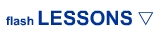ADVANCED/GAME Lesson 1: Control Movieclips Lesson 2: Flip Symbol Lesson 3: Inside Movieclip Lesson 4: Dynamic Text Lesson 5: Random Clip Lesson 6: Actionscript Music Lesson 7: Symbol Timer Lesson 8: Rotate Movieclip Lesson 9: Disable Right-click Lesson 10: Frame Names Lesson 11: Side Scroller Lesson 12: Increasing Velocity Lesson 13: Visibility Lesson 14: What's 'this' Lesson 15: Collectable Items Lesson 16: Specified Action Lesson 17: Button Tap Code Lesson 18: Random in Detail Lesson 19: Health Bar Lesson 20: Follow Movieclips MORE GAMES Lesson 1: Movement Lesson 2: Wall Collision Lesson 3: Shoot Lesson 4: Shot Collision Lesson 5: Score ANIMATION IN FLASH Lesson 1: Character Lesson 2: Eye Movement Lesson 3: Arm Movement Lesson 4: Walking Lesson 5: Background Lesson 6: Loop Background ROB's CORNER Introduction Lesson 1: Orbital Dots 1 Lesson 2: Orbital Dots 2 Lesson 3: Spinning 1 Lesson 4: Spinning 2 Lesson 5: Easing Spaceship Lesson 6: Sprite 3d Lesson 7: Bouncy Ball Lesson 8: Bouncy Ball 2 2 Lesson 9: Simple Collision Lesson 10: Crazy Tiles ****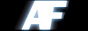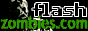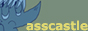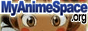Link Us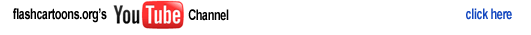home > learn flash > advanced lesson 18

Advanced Lesson Eighteen: Random in detail

 1.) In this tutorial I will teach you a little bit more of how the random function works in a little bit more detail. --------- --------------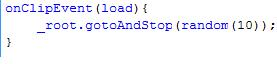--------- -------------- 2.) In this tutorial You need the following: -Actions Panel -Timeline if you want to test3.) This is what a random function looks like. This random function says go to a random frame, and then it has a parameter of 10. --------- ----------------------- -------------- 4.) How ever if you notice, when you test this code. The random action will never take you to the 10th frame by random. This is because the random function includes the number 0 as a number, so when you say random(10), you are really saying go to a random number between 0-9. whuch if you include 0 and count to 9, that is 10 numbers, but there is no 0th frame. --------- --------------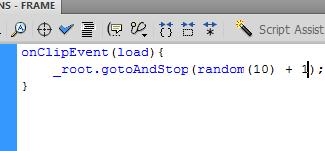--------- -------------- 5.) To fix this you simplely add one number to the outcome of random like so. --------- ----------------------- -------------- 6.) Then when executed it will be possible to make it to the 10th frame by random, because it adds 1 to each random number, and if the random number was 0, the frame number it will go to is that number plus 1, which would be frame one, and so if the random number was 9, it would go to frame 10. -jordan